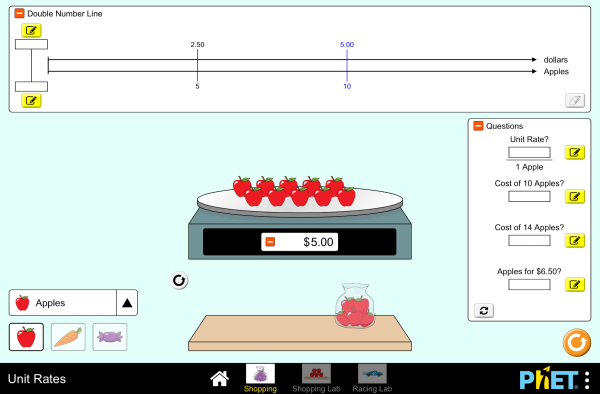Unit Rates_單位比例下載嵌入 關閉 嵌入模擬教學執行複本 利用 HTML 來嵌入模擬教學執行複本。您可以在 HTML 中改變已嵌入模擬教學的寬度及高度。 嵌入啓動模擬教學之圖樣

標題

• Ratios
• Proportional Reasoning
• Double Number Line
• Unit Rate

描述

Discover the unit rate while shopping for fruits, vegetables, and candy. Construct a double number line and look for patterns. Challenge yourself on the race track as you compare cars with different rates!

HTML5 版本之翻譯：

學習目標

• Interpret ratios of mixed units (e.g. \$/lb)
• Define the unit rate and determine a method to calculate it
• Predict how changing the numerator or denominator of a rate will affect the unit rate
• Use the double number line to reason about rates and solve real-world problems
• Develop strategies to use the unit rate to solve problems
• Compare unit rates between two simultaneous situations

標準對齊

共用核心 - 數學

6.RP.A.2
Understand the concept of a unit rate a/b associated with a ratio a:b with b ≠ 0, and use rate language in the context of a ratio relationship. For example, "This recipe has a ratio of 3 cups of flour to 4 cups of sugar, so there is 3/4 cup of flour for each cup of sugar." "We paid \$75 for 15 hamburgers, which is a rate of \$5 per hamburger."1
6.RP.A.3
Use ratio and rate reasoning to solve real-world and mathematical problems, e.g., by reasoning about tables of equivalent ratios, tape diagrams, double number line diagrams, or equations.
6.RP.A.3b
Solve unit rate problems including those involving unit pricing and constant speed. For example, if it took 7 hours to mow 4 lawns, then at that rate, how many lawns could be mowed in 35 hours? At what rate were lawns being mowed?
6.RP.A.3d
Use ratio reasoning to convert measurement units; manipulate and transform units appropriately when multiplying or dividing quantities.

教學提示模擬教學的控制概觀、模型簡化與引導學生思考 ( PDF ).

老師提供的活動

Preguntas de razonamiento para todas las simulaciones HTML5Diana López 大學-簡介

K-5

Finding and Using Unit RatesKarina K. R. Hensberry 國中 指引 數學
Using Ratios and Rates to Solve ProblemsIan Whitacre, Sebnem Tekin 國中 指引 數學
Comparing Unit RatesIan Whitacre, Sebnem Tekin 國中 指引 數學
Grocery ShoppingDaniel Greenberg 國中 指引 數學
Middle School Math Sim AlignmentAmanda McGarry 國中 其它 數學
Mapping of PhET and IBDP Physics Jaya Ramchandani 高中 其它 物理
Using Unit Rate to Understand Slope Karissa Gonzalez 國中

Finding Unit Rates Using Addition, Subtraction, Multiplication, and Division Bethany Konz 國中 指引 數學

Serbian 全部 СрпскиПијаца, шопинг а и трка
Tamil 全部 Tamilஅலகின் விலைகள்

The new HTML5 sims can run on iPads and Chromebooks, as well as PC, Mac, and Linux systems.

iOS 11+ Safari

Android:
Not officially supported. If you are using the HTML5 sims on Android, we recommend using the latest version of Google Chrome.

Chromebook:
The HTML5 and Flash PhET sims are supported on all Chromebooks.
Chromebook compatible sims

Windows Systems:

Macintosh Systems:
macOS 10.9.5+, Safari 9+, latest version of Chrome.

Linux Systems:

• Amy Rouinfar (lead designer)
• Chris Malley (developer)
• Dave Schmitz (developer)
• Amy Hanson
• Amanda McGarry
• Susan Miller
• Ariel Paul
• Kathy Perkins
• almond-0.2.9.js
• base64-js-1.2.0.js
• easing-equations-r12
• FileSaver-b8054a2.js
• font-awesome-4.5.0
• game-up-camera-1.0.0.js
• jama-1.0.2
• jquery-2.1.0.js
• lodash-4.17.4.js
• pegjs-0.7.0.js
• seedrandom-2.4.2.js
• text-2.0.12.js
• TextEncoderLite-3c9f6f0.js
• Tween-r12.js